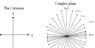# A difficult limited

m_s_a
When we think a value an end in the compound functions :
We remember that we in front of number from not a limited from the paths

#### Attachments

• lim(z)par.bmp
173.2 KB · Views: 486

Homework Helper
We remember that we in back of path from not a limited from the numbers. Is that wrong? I really can't understand you. Sorry.

m_s_a
We remember that we in back of path from not a limited from the numbers. Is that wrong? I really can't understand you. Sorry.

He is not at the end a presence
Why ؟
The previous way the others is suitable ? why ?...

#### Attachments

•Plane.JPG
22.7 KB · Views: 374
Homework Helper
He is not at the end a presence
Why ؟
The previous way the others is suitable ? why ?...

I will. When it's approved. I think that gives me time to get a good night's sleep.

Homework Helper
He is not at the end a presence
Why ؟
The previous way the others is suitable ? why ?...

Now I don't understand what your picture is trying to tell me either. The proof of the limit in your first post is just fine.

m_s_a
Now I don't understand what your picture is trying to tell me either. The proof of the limit in your first post is just fine.

I did not understand your intention ?
I do not have a confirmation of the response
But look at the next example:
I apologize
Second : on the axis z = y^2

#### Attachments

Last edited:
Homework Helper
You have now posted 3 different pictures that appear to involve three different problems. As Dick told you before, the first is correct. This third one is also correct- except for spelling: since you get two different limits by approaching (0,0) along two different paths, the limit does not exist.

I'm not sure what the second picture was supposed to represent. But one thing you should be careful about: The line y= 2x, for example, in R2, is not in the complex plane.

m_s_a
You have now posted 3 different pictures that appear to involve three different problems. As Dick told you before, the first is correct. This third one is also correct- except for spelling: since you get two different limits by approaching (0,0) along two different paths, the limit does not exist.
My answer of the first question :
is correct or a mistake?

I'm not sure what the second picture was supposed to represent. But one thing you should be careful about: The line y= 2x, for example, in R2, is not in the complex plane.

My answer of the first question :
is correct or a mistake?

remember:
x=(z+z')/2
y=(z-z')/2i
z'=z par

#### Attachments

• limm.bmp
215.1 KB · Views: 477
Homework Helper
lim(z->0) is not the same as lim(x->0) or lim(y->0). It's lim(x->0 AND y->0).

m_s_a
lim(z->0) is not the same as lim(x->0) or lim(y->0). It's lim(x->0 AND y->0).

Look into the proof of Coshi theory - Riman

f'(z)=f'(x)=f'(y)

no f'(z)=f'(x)+f'(y)

Homework Helper
Look into the proof of Coshi theory - Riman

f'(z)=f'(x)=f'(y)

no f'(z)=f'(x)+f'(y)

What have derivatives got to do with it? You were posting limit problems. Or something. I give up. I don't know what your question or problem is.

m_s_a
I support the second solution
And I am not sure